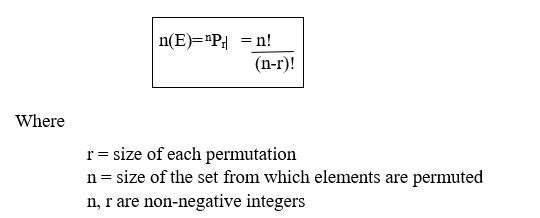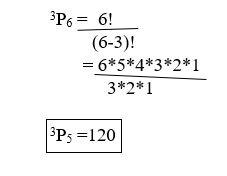### Permutation

• date 25th June, 2019 |
• by Prwatech |

# Permutation tutorial

Permutation Tutorial,  Here one can learn how to calculate the permutation in data science with example. Before we proceed, we recommend you to go through the previous blog in this series on the probability distribution tutorial.

If you are the one who is keen to learn the Data science then learn advanced Data science course from the best Data science training institute in Bangalore who can help guide you on the course from the 0 Level to Advanced level.

The Below mentioned permutation tutorial will help you to understand the detailed information about Permutation in Data science, so just follow all the tutorials of India’s Leading Data science training institute and Be a Pro Data analyst.

## What is permutation?

A Permutation is an arrangement of a number of objects in a definite order taken some.

### Why use permutation?

When we need to determine some items from a collection in a particular order then we use Permutation.

Like if we need to find Eight cousins should be accommodated in two 3-bed and one 2-bed room. In how many ways can they be accommodated?

### Permutation Formula:### Permutation example:

In how many ways we can pick if three T-Shirts are to be chosen from Red, Blue, Green, Orange, Yellow and Purple color without repetition.In this Permutation tutorial, we have covered concepts of what is a permutation in data science, permutation formula and how to calculate permutation with examples. This will get you a clear idea about Permutation in Data Science.

Interested in learning more? then get enroll with Prwatech for advanced Data science training in Bangalore with 100% job assistance.